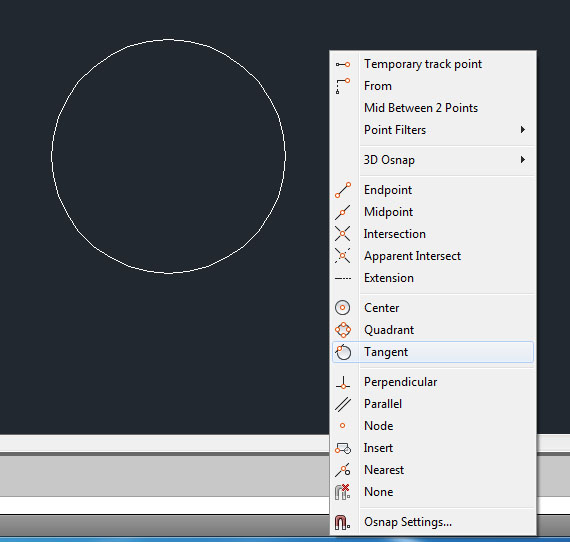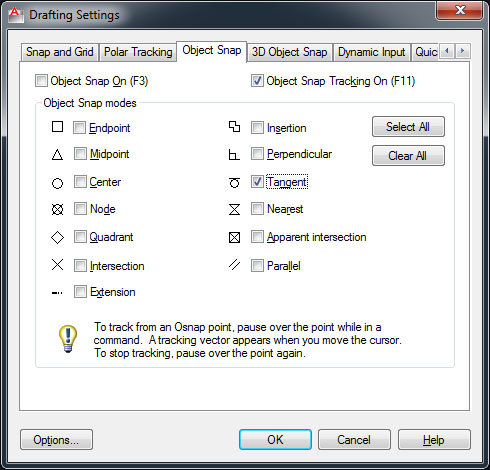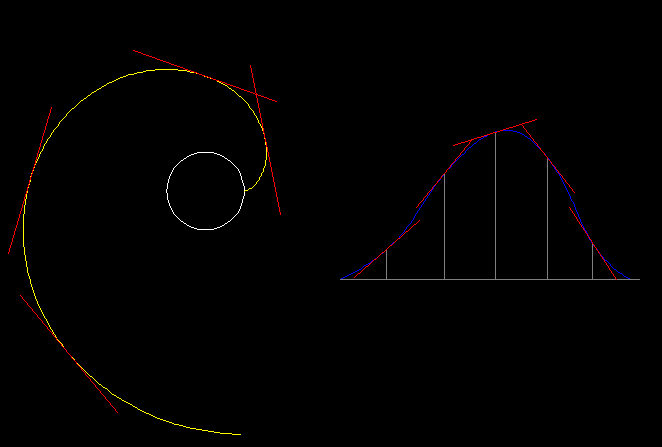# Accurate involute.## Recommended PostsI am drawing a gear and now i need to connect points to draw involute for a gear tooth:

http://i46.tinypic.com/1o6fls.png

Points are numbered. I'm trying to draw with a spline, but i don't know where to put last point of a spline to draw an exact involute - like for example - if i would draw like this and press enter - i would get this spline:

http://i46.tinypic.com/546ttv.png

But if i would move cursor in a different direction and then press enter - i would still get almost identical spline, but still, not the same:

http://i48.tinypic.com/2le1c3m.png

So how do i draw an accurate involute? Maybe i should draw one more point and then stop drawing spline?

Edited by Lurking
##### Share on other sitesIf you got the points from a formula, then yes, you can calculate more points at the top to increase the precision.

About the spline curves: if you move a point, the curve will get a different shape. The more far from the changed point, the more smaller the change. Theoretically, the whole curve will change but using enough points, the amount of change can be small enough.

My advice: use an additional point at the top to end the curve, and one more point (calculated with the same formula, of course) as tangent direction.

##### Share on other sitesThanks.

Well, i'm doing involute by the book - it's kinda mix of formula and graphical approach, so i just calculated couple more points and got that spline. Sadly enough, something went wrong and my gears..don't fit.

Another minor question - how can i draw tangent line to some point on the spline? Like that:

http://i47.tinypic.com/33lo5xc.png

I've read here that you can do this with offsetting - but that's not accurate enough, because from a graph i'll need to calculate some unknowns. Onsnapping not actually works too.

##### Share on other sitesYou can use the Tangent snap. Create a circle (any size) the start the line command. Pick anywhere on the screen and the hold down the shift key on the keyboard and right click with the mouse. You should get a floating menu with snap options on it. Choose the tangent snap and pick somewhere on the circle. The line should now be tangential to the circle. If the floating menu doesn't appear when you shift right click then type osnap at the command line and tick the box for tangent snap so it is on all the time. You can also draw a line tangential to two circles. With two circles present in your drawing, start the line command and (assuming you have the tangent snapof permanently) click on the first circle. Nothing will happen yet as this is a deferred snap and is waiting for a second point. click on the second circle and the line with be tangential to both circles.##### Share on other sitesI know how to do it in the circle, but the thing is - i need to do it on a spline. I have graph like this:

http://i45.tinypic.com/1zgu5q0.png

Its points, connected with a spline. Now i need for each point to have it's tangent:

http://i50.tinypic.com/104i5nt.png

And with snaps results are like this(i've chose tangent with right shift, and then pressed on a point):

http://i49.tinypic.com/dd0nm.png

So maybe i'm not using snaps correctly, or maybe someone has an idea how to do it?

##### Share on other sitesLurking,

this will draw a spline (I hope is the correct profile for gear)

```(defun c:gear (/ e o r a i l)
(if
(and
(setq e (car (entsel)))
(eq (cdr (assoc 0 (setq e (entget e)))) "CIRCLE")
)
(progn
(setq o (cdr (assoc 10 e))
r (cdr (assoc 40 e))
a (/ pi 180)
i -1)
(repeat 360
(setq l (cons (polar (polar o (* a (setq i (1+ i))) r) (- (* a i) (/ pi 2)) (* a i r)) l))
)
(entmake
(append
(list
'(0 . "SPLINE")
'(100 . "AcDbEntity")
'(100 . "AcDbSpline")
'(70 . 40)
'(71 . 3)
(cons 74 (length l))
'(44 . 1.0e-010)
)
(mapcar '(lambda (x) (cons 11 x)) l)
)
)
)
)
(princ)
)```

And this will draw tangent line to a curve

```(defun c:tgl (/ vs unit e p l d)
(defun unit (l / d)
(if (> (setq d (distance '(0 0 0) l)) 0.0)
(mapcar '(lambda (x) (/ x d)) l)
)
)

(defun vs (v s)
(mapcar '(lambda (x) (* x s)) v)
)

(if
(and
(setq e (ssget "_:E:S" '((0 . "*POLYLINE,SPLINE,ARC,CIRCLE,ELLIPSE"))))
(setq e (ssname e 0))
(not (redraw e 3))
(setq p (getpoint "\nSelect point on object: "))
(equal p (setq p (vlax-curve-GetClosestPointTo e p)) 1e-10)
(not (redraw e 4))
)
(progn
(setq l (* 0.1 (vlax-curve-GetDistAtParam e (vlax-curve-GetEndParam e)))
d (vs (unit (vlax-curve-GetFirstderiv e (vlax-curve-GetParamAtPoint e p))) l)
)
(entmake
(list
'(0 . "line")
(cons 10 (mapcar '- p d))
(cons 11 (mapcar '+ p d))
)
)
)
)
(princ)
)```First one is drawing a complete curve, as it travel thru entire circle. Obviously, you don't need it that way.

The second will draw a line which has length equal 20% of selected curve, but it can be adjusted.

## Join the conversation

You can post now and register later. If you have an account, sign in now to post with your account.
Note: Your post will require moderator approval before it will be visible.×   Pasted as rich text.   Restore formatting

Only 75 emoji are allowed.